Smoothing is used to reduce noise or to produce a less pixelated image. Most smoothing methods are based on low-pass filters, but you can also smooth an image using an average or median value of a group of pixels (a kernel) that moves through the image.

The following example uses the SMOOTH and MEDIAN functions with a moving average on a photomicrograph image of human red blood cells. This example data is available in the examples/data directory of your IDL installation.

The code shown below creates the following images, each displayed in separate windows.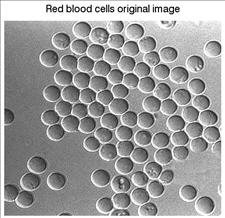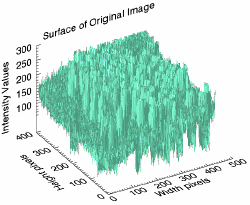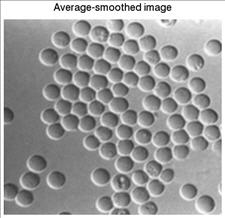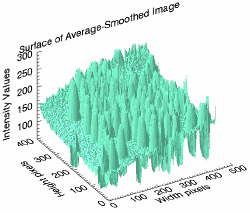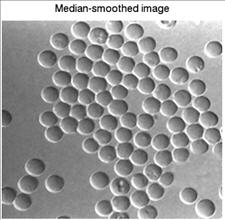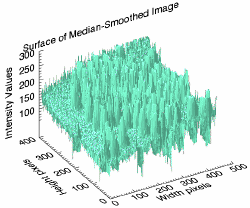Copy the entire code block and paste it into the IDL command line to run it.

`; Select the file.`
`file = FILEPATH('rbcells.jpg', \$`
`   SUBDIRECTORY = ['examples', 'data'])`
` `
`; Use READ_JPEG to define the image as byte data for `
`; the SURFACE function.`
`READ_JPEG, file, rbcimage`
` `
`; Display the image.`
`img01 = IMAGE(rbcimage, LOCATION = [0, 0], \$`
`   TITLE = 'Red blood cells original image')`
` `
`; Display the image as a 3D surface. The resulting image shows `
`; many discontinuous values as sharp spikes in the middle range `
`; of values.`
`s1 = SURFACE(rbcimage, LOCATION = [500, 0], \$`
`   XTITLE = 'Width pixels', \$`
`   YTITLE = 'Height pixels', \$`
`   ZTITLE = 'Intensity Values', \$`
`   TITLE = 'Surface of Original Image', \$`
`   COLOR = 'aquamarine', \$`
`   ZTICKVALUES = [100, 150, 200, 250, 300])`
`; move the z-axis to the back`
`(s1['zaxis']).location = [0, (s1.yrange), 0] `
` `
`; Smooth the image with the SMOOTH function, which uses the`
`; average value of a moving 5 x 5 kernel applied to each pixel `
`; in the image.`
`smoothed_image = SMOOTH(rbcimage, 5, /EDGE_TRUNCATE)`
` `
`; Display the average-smoothed image.`
`img02 = IMAGE(smoothed_image, LOCATION = [0, 50], \$`
`   TITLE = 'Average-smoothed image')`
` `
`s2 = SURFACE(smoothed_image, location = [500, 50], \$`
`   XTITLE = 'Width pixels', \$`
`   YTITLE = 'Height pixels', \$`
`   ZTITLE = 'Intensity Values', \$`
`   TITLE = 'Surface of Average-Smoothed Image', \$`
`   COLOR = 'aquamarine', \$`
`   ZTICKVALUES = [100, 150, 200, 250, 300])`
`; move the z-axis to the back`
`(s2['zaxis']).location = [0, (s2.yrange), 0]`
` `
`; Smooth the image with the MEDIAN function, which uses the`
`; median value of a moving 5 x 5 kernel applied to each pixel `
`; in the image.`
`median_image = MEDIAN(rbcimage, 5)`
` `
`; Display the median-smoothed image.`
`img03 = image(median_image, LOCATION = [0, 100], \$`
`   TITLE = 'Median-smoothed image')`
` `
`s3 = SURFACE(median_image, LOCATION = [500, 100], \$`
`   XTITLE = 'Width pixels', \$`
`   YTITLE = 'Height pixels', \$`
`   ZTITLE = 'Intensity Values', \$`
`   TITLE = 'Surface of Median-Smoothed Image', \$`
`   COLOR = 'aquamarine', \$`
`   ZTICKVALUES = [100, 150, 200, 250, 300])`
`; move the z-axis to the back`
`(s3['zaxis']).location = [0, (s3.yrange), 0]`